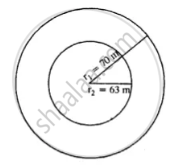# The Radii of the Inner and Outer Circumferences of a Circular Running Track Are 63 M and 70 M Respectively. Find : (I) the Area of the Track ; (Ii) the Difference Between the Lengths of the Two. - Mathematics

Sum

The radii of the inner and outer circumferences of a circular running track are 63 m and 70 m respectively. Find :
(i) the area of the track ;
(ii) the difference between the lengths of the two circumferences of the track.

#### Solution∴ Area of track = pir_1^2 - pir_2^2

= 22/7[(70)^2 - (63)^2]

= 22/7(70 + 63)(70 - 63)

= 22/7 xx 133 xx 7

= 2926 m2

Length of outer edge i.e. circumference

= 2pir_1

= 2 xx 22/7 xx 70 = 440m

Length of inner edge = 2pir_2

= 2 xx 22/7 xx 63 = 396 m

Difference between lengths of two circumferences = 440 – 396 = 44 m
Hence (i) 2926 m2 (ii) 44 m

Concept: Area of Circle
Is there an error in this question or solution?

#### APPEARS IN

Selina Concise Mathematics Class 8 ICSE
Chapter 20 Area of a Trapezium and a Polygon
Exercise 20 (D) | Q 7 | Page 234